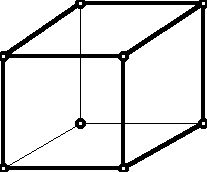Mathematics Research Projects

Problem Set III

Prepared by:

Joseph Malkevitch
Department of Mathematics and Computing
York College (CUNY)
Jamaica, New York 11451-0001

Email: malkevitch@york.cuny.edu (for additions, suggestions, and corrections)

web page: http://york.cuny.edu/~malk/

Comments and insights into these questions are welcomed and should be sent to the email address above.

Problem 1

Hidden Line Representations of Convex-Polytopes

Prepared by:

Joseph Malkevitch
Department of Mathematics and Computing
York College (CUNY)
Jamaica, New York 11451-0001

email: malkevitch@york.cuny.edu
web page: http://york.cuny.edu/~malk/

A traditional way to represent 3-dimensional convex polyhedra on a flat piece of paper is to use a hidden line representation:Usually hidden lines are shown as dotted lines but above I have used thin lines for the hidden lines. Note that one is interested in drawings where all of the vertices, edges, and faces of the polyhedron are shown.

If one starts with a square pyramid and truncates the one vertex where four triangles meet, one gets a truncated (square) pyramid. This polyhedron is combinatorially equivalent to the cube. The "usual" drawing of this solid also has three hidden lines.

Here are some questions to investigate with regard to hidden line representations of convex 3-dimensional polyhedra in the plane.

Question 1:

a. For a fixed convex polyhedron P, what are the largest number and smallest number of hidden lines that can occur in a drawing of P in the plane?

b. For a fixed convex polyhedron P, what are the largest number and smallest number of hidden lines that can occur in a drawing of a polyhedron combinatorially equivalent to P in the plane?

Question 2:

Address the questions above for special classes of 3-dimensional polyhedra:

a. those where all the vertices have the same valence (number of edges at a vertex) 3.

b. those where all the vertices have the same valence 4

c. those where all the vertices have the same valence 5

d. those were all the faces are triangles

e. those where all of the faces have four sides

f. those where all the face have five sides.

Comment: The interesting problem seems to be to determine the minimum number of of hidden lines for all the different drawings of polyhedra in the same combinatorial type of polyhedron P.